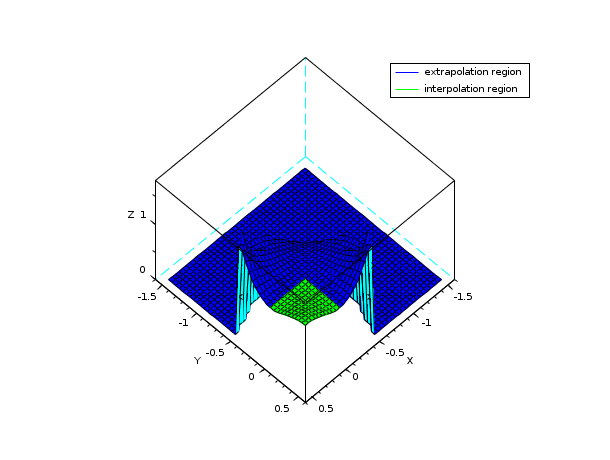Scilab Home page | Wiki | Bug tracker | Forge | Mailing list archives | ATOMS | File exchange
Change language to: English - Français - Português - 日本語 -

See the recommended documentation of this function

Справка Scilab >> Interpolation > eval_cshep2d

# eval_cshep2d

bidimensional cubic shepard interpolation evaluation

### Calling Sequence

`[zp [,dzpdx, dzpdy [,d2zpdxx,d2zpdxy,d2zpdyy]]] = eval_cshep2d(xp, yp, tl_coef)`

### Arguments

xp, yp

two real vectors (or matrices) of the same size

tl_coef

a tlist scilab structure (of type cshep2d) defining a cubic Shepard interpolation function (named `S` in the following)

zp

vector (or matrix) of the same size than `xp` and `yp`, evaluation of the interpolant `S` at these points

dzpdx,dzpdy

vectors (or matrices) of the same size than `xp` and `yp`, evaluation of the first derivatives of `S` at these points

d2zpdxx,d2zpdxy,d2zpdyy

vectors (or matrices) of the same size than `xp` and `yp`, evaluation of the second derivatives of `S` at these points

### Description

This is the evaluation routine for cubic Shepard interpolation function computed with cshep2d, that is :### Remark

The interpolant S is C2 (twice continuously differentiable) but is also extended by zero for (x,y) far enough the interpolation points. This leads to a discontinuity in a region far outside the interpolation points, and so, is not cumbersome in practice (in a general manner, evaluation outside interpolation points (i.e. extrapolation) leads to very inaccurate results).

### Examples

```// see example section of cshep2d

// this example shows the behavior far from the interpolation points ...
deff("z=f(x,y)","z = 1+ 50*(x.*(1-x).*y.*(1-y)).^2")
x = linspace(0,1,10);
[X,Y] = ndgrid(x,x);
X = X(:); Y = Y(:); Z = f(X,Y);
S = cshep2d([X Y Z]);
// evaluation inside and outside the square [0,1]x[0,1]
m = 40;
xx = linspace(-1.5,0.5,m);
[xp,yp] = ndgrid(xx,xx);
zp = eval_cshep2d(xp,yp,S);
// compute facet (to draw one color for extrapolation region
// and another one for the interpolation region)
[xf,yf,zf] = genfac3d(xx,xx,zp);
colors = 2*ones(1,size(zf,2));
// indices corresponding to facet in the interpolation region
ind=find( mean(xf,"r")>0 & mean(xf,"r")<1 & mean(yf,"r")>0 & mean(yf,"r")<1 );
colors(ind)=3;
clf();
plot3d(xf,yf,list(zf,colors), flag=[2 6 4])
legends(["extrapolation region","interpolation region"],[2 3],1)
show_window()```• cshep2d — bidimensional cubic shepard (scattered) interpolation

### History

 Версия Описание 5.4.0 previously, imaginary part of input arguments were implicitly ignored.